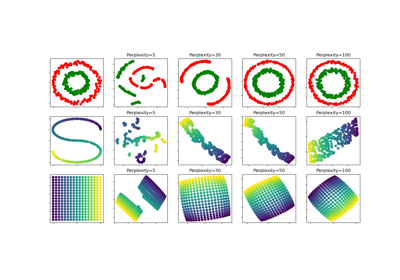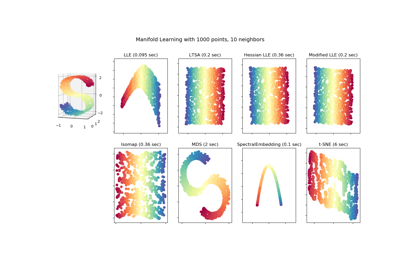# sklearn.datasets.make_s_curve¶

sklearn.datasets.make_s_curve(n_samples=100, noise=0.0, random_state=None)[source]

Generate an S curve dataset.

Read more in the User Guide.

Parameters: n_samples : int, optional (default=100) The number of sample points on the S curve. noise : float, optional (default=0.0) The standard deviation of the gaussian noise. random_state : int, RandomState instance or None (default) Determines random number generation for dataset creation. Pass an int for reproducible output across multiple function calls. See Glossary. X : array of shape [n_samples, 3] The points. t : array of shape [n_samples] The univariate position of the sample according to the main dimension of the points in the manifold.

## Examples using sklearn.datasets.make_s_curve¶t-SNE: The effect of various perplexity values on the shapeComparison of Manifold Learning methods